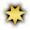View Single PostCMoray
 01.22.2018 , 01:22 PM | #30 Quote Quote: Originally Posted by EvolixeHow do you get to 2700 crit? Check your math again. 8 x Enhancement = 8 x 231 = 1848 6 x Augment = 6 x 98 = 588 2 x Relic = 2 x 66 = 132 2 x Crystal = 2 x 41 = 82 1 x Stim = 1 x 99 = 99 => 1848 + 588 + 132 + 82 + 99 = 2749Aurinko